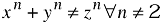# equation

\$Revision: 5565 \$

\$Date: 2006-02-16 08:50:26 -0500 (Thu, 16 Feb 2006) \$

equation — A displayed mathematical equation

## Synopsis

### Content Model

```equation ::=
(`blockinfo`?,
(`title`,`titleabbrev`?)?,
(`informalequation`|
(`alt`?,
(`graphic`+|`mediaobject`+|`mathphrase`+))))```

### Attributes

Common attributes

 Name Type Default floatstyle CDATA None label CDATA None

## Description

An `Equation` is a formal mathematical equation (with an optional rather than required title).

If the MathML Module is used, `Equation` can also contain the `mml:math` element.

### Processing expectations

Formatted as a displayed block. For an inline equation, use `InlineEquation`.

Processing systems that number equations or build a table of equations at the beginning of a document may have difficulty correctly formatting documents that contain both `Equation`s with `Title`s and `Equation`s without `Title`s. You are advised to use `InformalEquation` for equations without titles.

### Future Changes

In some future version of DocBook, probably V5.0, even though the change has not yet been announced, the `Title` on `Equation` will be required. For equations without titles, use `InformalEquation`.

### Children

The following elements occur in equation: .

## Attributes

label

`Label` specifies an identifying string for presentation purposes.

Generally, an explicit `Label` attribute is used only if the processing system is incapable of generating the label automatically. If present, the `Label` is normative; it will used even if the processing system is capable of automatic labelling.

## Examples

```<!DOCTYPE equation PUBLIC "-//OASIS//DTD DocBook XML V4.1.2//EN"
"http://www.oasis-open.org/docbook/xml/4.1.2/docbookx.dtd">
<equation><title>Fermat's Last Theorem</title>
<alt>x^n + y^n &ne; z^n &forall; n &gt; 2</alt>
<graphic fileref="figures/fermat.png"/>
</equation>```

Equation 1. Fermat's Last TheoremAlternatively, for relatively simple equations such as Fermat's Last Theorem, `mathphrase` is often sufficient:

```<!DOCTYPE equation PUBLIC "-//OASIS//DTD DocBook XML V4.5//EN"
"http://www.oasis-open.org/docbook/xml/4.5/docbookx.dtd">
<equation><title>Fermat's Last Theorem</title>
<alt>x^n + y^n &ne; z^n &forall; n &gt; 2</alt>
<mathphrase>x<superscript>n</superscript>
+ y<superscript>n</superscript>
≠ z<superscript>n</superscript>
∀ n ≠ 2</mathphrase>
</equation>```

Equation 2. Fermat's Last Theorem

xn + yn ≠ zn ∀ n ≠ 2